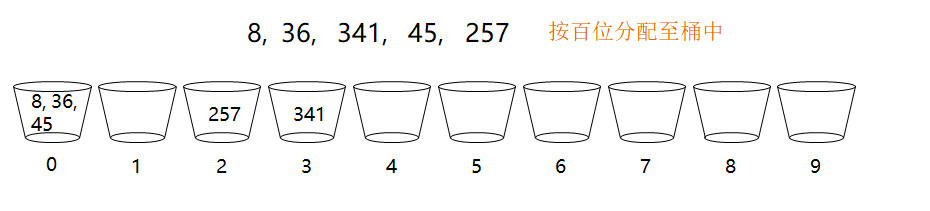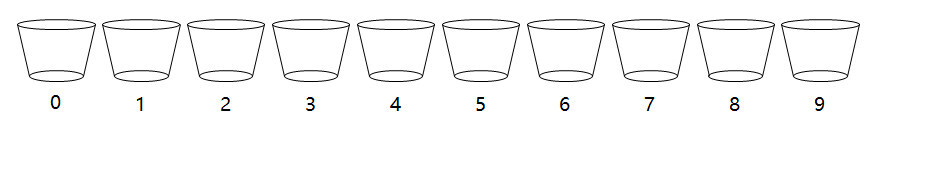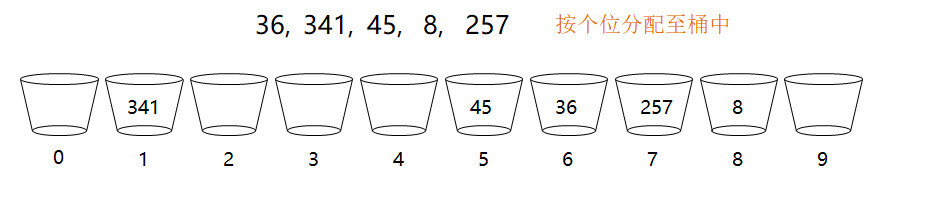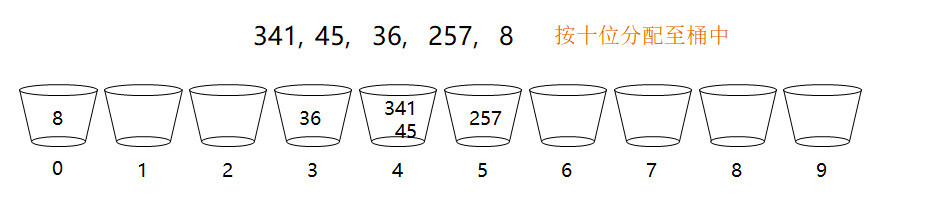# 手写基数排序算法1. 计算出待排序数列的最大值 max

2. 计算出最大值的最高位位数 div_cnt

3. 分配内存，代表 0~9 的 10 个桶，用于存储数据

4. 循环 div_cnt 次，每次根据一个分位(个位,十位,百位...)，将这些数据分配到桶中，然后再按桶的顺序将数据收集回来。循环结束后，数据就已经是排好序的了。

5. 释放内存。(ivec_t 是动态变长数组，存储 integer 数据。类似 c++中的 vector，代码实现见后面)

void radix_sort(int* parr, int count) {    // 1. compute maximum value    int max = parr;    for (int i = 1; i < count; i++) {        if (parr[i] > max) {            max = parr[i];        }    }    // 2. computer div_cnt    int div_cnt = 0;    int max_temp = max;    while (max_temp > 0) {        max_temp /= 10;        div_cnt++;    }    // 3. alloc memory for sorting    ivec_t* pvecs = (ivec_t*)malloc(10 * sizeof(ivec_t));    for (int i = 0; i < 10; i++) {        ivec_init(pvecs + i, 8);    }    // 4. sort    int divisor = 1;    for (int k = 0; k < div_cnt; k++) {        // clear memory        for (int i = 0; i < 10; i++) {            ivec_clear(pvecs + i);        }        // distribute to ivec        for (int i = 0; i < count; i++) {            int idx = (parr[i] / divisor) % 10;            ivec_append(pvecs + idx, parr[i]);        }        divisor *= 10;        // collect from ivec        int idx = 0;        for (int i = 0; i < 10; i++) {            int* pbuf = ivec_buf(pvecs + i);            int cnt = ivec_len(pvecs + i);            for (int n = 0; n < cnt; n++) {                parr[idx++] = pbuf[n];            }        }    }    // 5. free memory    for (int i = 0; i < 10; i++) {        ivec_destroy(pvecs + i);    }    free(pvecs);}

typedef struct ivec_t {    int cap;    int len;    int* pbuf;} ivec_t;int ivec_init(ivec_t* pvec, int cap);int ivec_append(ivec_t* pvec, int val);int ivec_len(ivec_t* pvec);int* ivec_buf(ivec_t* pvec);void ivec_clear(ivec_t* pvec);int ivec_destroy(ivec_t* pvec);int ivec_init(ivec_t* pvec, int cap) {    pvec->len = 0;    pvec->cap = cap;    pvec->pbuf = (int*)malloc(cap * sizeof(int));    return 0;}int ivec_append(ivec_t* pvec, int val) {    if (pvec->len >= pvec->cap) {   // need expand        int new_cap = pvec->cap * 2;        int* pnew_buf = realloc(pvec->pbuf, new_cap * sizeof(int));        if (!pnew_buf) {            return -1;        }        pvec->pbuf = pnew_buf;        pvec->cap = new_cap;    }    pvec->pbuf[pvec->len++] = val;    return 0;}int ivec_len(ivec_t* pvec) {    return pvec->len;}int* ivec_buf(ivec_t* pvec) {    return pvec->pbuf;}void ivec_clear(ivec_t* pvec) {    pvec->len = 0;}int ivec_destroy(ivec_t* pvec) {    free(pvec->pbuf);    memset(pvec, 0, sizeof(*pvec));    return 0;}

​我的微信号是 实力程序员，欢迎大家转发至朋友圈，分享给更多的朋友。## 评论